# Trick to calculate determinant of a 3×3 matrix

• Last Updated : 14 Aug, 2020

Linear Algebra is one of the most important topics in Engineering Mathematics Gate syllabus. Finding determinant of a matrix is one of the most important problems in Linear Algebra. Finding Determinant of a matrix is required for finding inverse of a matrix, determining whether vectors are linearly independent or not etc.

Let us consider a matrix and its determinant be A, then A can be calculated as given below.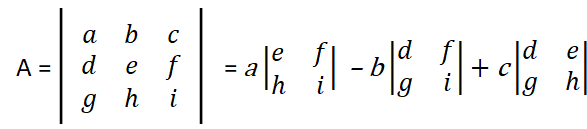where,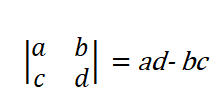Example :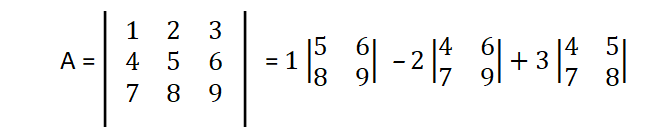```A = 1( 5*9 – 6*8) – 2(4*9 – 6*7) + 3(4*8 – 5*7)
A = 1(45 – 48) – 2(36 – 42) + 3(32 – 35)
A = 1*(-3) – 2*(-6) + 3*(-3)
A = -3 + 12 – 9
A = 0 ```

The above traditional method consumes a lot of time especially when you are solving some complex problem. There is another easier and faster method determinant of a matrix. The below shown is faster way of solving the determinant of a matrix.

Another Method for faster calculation :
In this method we represent the matrix in different method only for determinant calculation.

Consider a matrix and convert it into the following given below.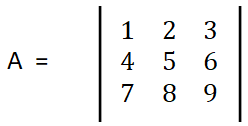write the matrix as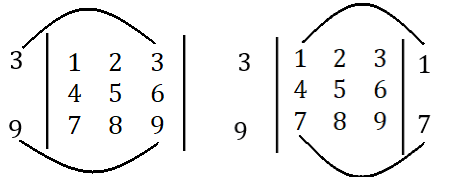Now perform the following operation,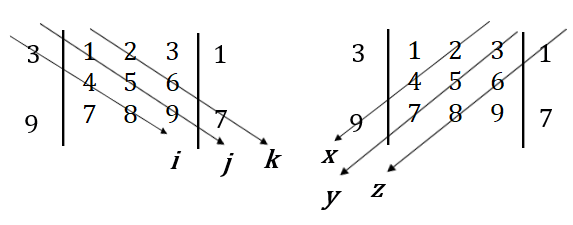Here,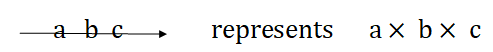So, from the above matrix, we can write,

```i = (3*4*8) = 96     x = (2*4*9) = 72
j = (1*5*9) = 45     y = (3*5*7) = 105
k = (2*6*7) = 84     z = (1*6*8) = 48

A = (i + j + k) – (x + y + z) = (96 + 45 + 84) – (72 + 105 + 48)
= (225 – 225)
= 0 ```

So, by following the above we can calculate the determinant of a matrix easily. It requires practice to change our method of calculation from traditional method to easy method but it is worth practicing,

Note –
This method works only for (3, 3) matrix.

My Personal Notes arrow_drop_up
Recommended Articles
Page :